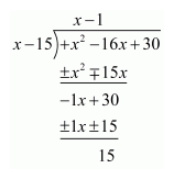# What should be subtracted to the polynomial x2 − 16x + 30,`
Question:

What should be subtracted to the polynomial $x^{2}-16 x+30$, so that 15 is the zero of the resulting polynomial?

(a) 30

(b) 14

(c) 15

(d) 16

Solution:

We know that, if $x=\alpha$, is zero of a polynomial then $x-\alpha$ is a factor of $f(x)$

Since 15 is zero of the polynomial $f(x)=x^{2}-16 x+30$, therefore $(x-15)$ is a factor of $f(x)$

Now, we divide $f(x)=x^{2}-16 x+30$ by $(x-15)$ we getThus we should subtract the remainder 15 from $x^{2}-16 x+30$,

Hence, the correct choice is (c)